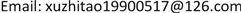OJNSOpen Journal of Nature Science2330-1724Scientific Research Publishing10.12677/OJNS.2019.73026OJNS-30305OJNS20190300000_26112298.pdf数学与物理 地球与环境 信息通讯 生命科学 化学与材料 流管法在水驱动态中的应用研究 Application of Stream Tube Method on Waterflooding Performance 志涛12null大庆油田第二采油厂，黑龙江 大庆290320190703180185© Copyright 2014 by authors and Scientific Research Publishing Inc. 2014This work is licensed under the Creative Commons Attribution International License (CC BY). http://creativecommons.org/licenses/by/4.0/Copyright © 2019 by author(s) and Hans Publishers Inc.1. 引言

2. 流管模型的建立与计算2.1. 流管模型的建立

A ( ξ ) = { 2 tan Δ α 2 h ⋅ ξ ( r w ≤ ξ ≤ L 2 ) 2 tan Δ α 2 h ⋅ ( L − ξ ) ( L 2 < ξ ≤ L − r w ) (1)

2.2. 流管模型中驱替过程的计算

p i w f − p w f = q ⋅ 1 K ∫ r w L − r w d ξ A ( ξ ) ( K r w μ w + K r o μ o ) (2)

R = 1 K ∫ r w L − r w d ξ A ( ξ ) ( K r w μ w + K r o μ o ) (3)

R = 1 K [ ∫ r w L f d ξ A ( ξ ) ( K r w μ w + K r o μ o ) + ∫ L f L − r w d ξ A ( ξ ) K r o ( S w c ) μ o ] (4)

2.3. 动态计算

Q w = 8 ∑ i = 1 N q w ( i ) (6)

R P = ∑ j = 1 T Δ t Q o ( j ) Δ t S total h ϕ ⋅ ( 1 − S w c ) (7)

3. 动态分析

Basic model parameter

4. 结论

1) 建立了计算水驱动态的计算方法，完善了油藏工程理论方法，它可以用来计算产油量、产水量以及采出程度。

2) 通过分析计算的水驱动态变化，并结合驱替前缘动态，指出流管中注入水的突破会影响动态结果。